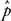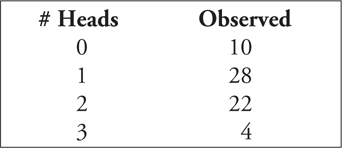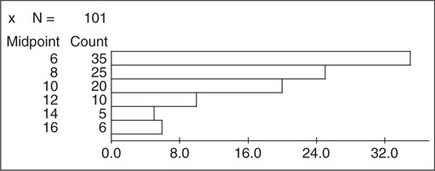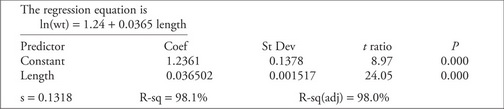# AP Statistics Practice Test 10

### Test Information10 questions23 minutes

1. A sports talk show asks people to call in and give their opinion of the officiating in the local basketball team's most recent loss. What will most likely be the typical reaction?

2. A major polling organization wants to predict the outcome of an upcoming national election (in terms of the proportion of voters who will vote for each candidate). They intend to use a 95% confidence interval with margin of error of no more than 2.5%. What is the minimum sample size needed to accomplish this goal?

3. A sample of size 35 is to be drawn from a large population. The sampling technique is such that every possible sample of size 35 that could be drawn from the population is equally likely. What name is given to this type of sample?

4. A teacher's union and a school district are negotiating salaries for the coming year. The teachers want more money, and the district, claiming, as always, budget constraints, wants to pay as little as possible. The district, like most, has a large number of moderately paid teachers and a few highly paid administrators. The salaries of all teachers and administrators are included in trying to figure out, on average, how much the professional staff currently earn. Which of the following would the teachers' union be most likely to quote during negotiations?

5. Alfred and Ben don't know each other but are each considering asking the lovely Charlene to the school prom. The probability that at least one of them will ask her is 0.72. The probability that they both ask her is 0.18. The probability that Alfred asks her is 0.6. What is the probability that Ben asks Charlene to the prom?

6. A significance test of the hypothesis H0: p= 0.3 against the alternative HA: p> 0.3 found a value of= 0.35 for a random sample of size 95. What is the P-value of this test?

7. Which of the following describe/s the central limit theorem?

I. The mean of the sampling distribution of x-is the same as the mean of the population.

II. The standard deviation of the sampling distribution of x-is the same as the standard deviation of x- divided by the square root of the sample size.

III. If the sample size is large, the shape of the sampling distribution of x- is approximately normal.

8. If three fair coins are flipped, P(0 heads) = 0.125, P(exactly 1 head) = 0.375, P(exactly 2 heads) = 0.375, and P(exactly 3 heads) = 0.125. The following results were obtained when three coins were flipped 64 times:What is the value of the X2statistic used to test if the coins are behaving as expected, and how many degrees of freedom does the determination of the P-value depend on?

9.For the histogram pictured above, what is the class interval (boundaries) for the class that contains the median of the data?

10. Thirteen large animals were measured to help determine the relationship between their length and their weight. The natural logarithm of the weight of each animal was taken and a least-squares regression equation for predicting weight from length was determined. The computer output from the analysis is given below:Give a 99% confidence interval for the slope of the regression line. Interpret this interval.